DCP HOME

# Quadratic L-Convex Minimization

For a symmetric matrix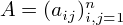with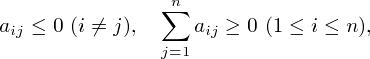the associated quadratic form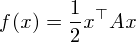is an L-convex function [1,2].

Here we consider a quadratic L-convex function: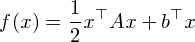.

In this web application, you can choose the dimension n from 1 to 7.

dimension n =

You can input parameters aij and bi in the ranges -5 ≤ aij≤ 0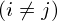, 0 < aii≤ 50 and -100 ≤ bi≤ 100. If the input does not satisfy the conditionthe unsatisfactory condition turns red.

You can also input an initial solution x.

 A 1 2 3 1 2 3

 1 2 3 b x

(Note: "Reset" button resets your inputs to default values.)

This web application minimizes f(x) using ODICON.

 K. Murota (2001): "Discrete Convex Analysis---An Introduction (in Japanese)," Kyoritsu Publishing Company, Tokyo. Section 5.2.

 K. Murota (2003): "Discrete Convex Analysis," SIAM. Section 7.3.